# 大数据线性回归预测学生成绩

## 1. 实验数据背景

### 1.2 数据简介

1.学校-学生学校（二进制：“ GP”-加布里埃尔·佩雷拉（Gabriel Pereira）或“ MS”-Mousinho da Silveira）

2.性别-学生的性别（二进制：“ F”-女性或“ M”-男性）

3.年龄-学生的年龄（数字：15至22）

4.地址-学生的家庭住址类型（二进制：“ U”-城市或“ R”-农村）

5.famsize-家庭大小（二进制：“ LE3”-小于或等于3或“ GT3”-大于3）

6.Pstatus-父母的同居状态（二进制：“ T”-同居或“ A”-分开）

7.Medu-母亲的教育（数字：0-无，1-初等教育（四年级），2 – 5至9年级，3 –中等教育或4 –高等教育）

8.Fedu-父亲的教育（数字：0-无，1-初等教育（四年级），2 – 5至9年级，3 –中等教育或4 –高等教育）

9.Mjob-母亲的工作（名义：“教师”，“与健康”有关的，民事“服务”（例如行政或警察），“在家”或“其他”）

10.Fjob-父亲的工作（名义：“教师”，“与健康”相关的，民事“服务”（例如行政或警察），“在家”或“其他”）

11.理由-选择这所学校的理由（名义：接近“家”，学校“声誉”，“课程”偏好或“其他”）

12.监护人-学生的监护人（名词：“母亲”，“父亲”或“其他”）

13.traveltime-学校到学校的旅行时间（数字：1-<15分钟，2-15至30分钟，3-30分钟至1小时或4-> 1小时）

14.学习时间-每周学习时间（数字：1-<2小时，2-2至5小时，3-5至10小时或4-> 10小时）

15.失败-过去类失败的次数（数字：如果1 <= n ❤️，则为n，否则为4）

16.schoolup-额外的教育支持（二进制：是或否）

17.famsup-家庭教育支持（二进制：是或否）

18.付费-课程主题内的额外付费课程（数学或葡萄牙语）（二进制：是或否）

19.活动-课外活动（二进制：是或否）

20.托儿所-上托儿所（二进制：是或否）

21.更高-想要接受高等教育（二进制：是或否）

22.互联网-在家上网（二进制：是或否）

23.浪漫-具有浪漫关系（二进制：是或否）

24.家族-家庭关系的质量（数字：从1-非常差到5-极好）

25.空闲时间-放学后的空闲时间（数字：从1-非常低到5-非常高）

26.外出-与朋友外出（数字：从1-非常低到5-非常高）

27.Dalc-工作日酒精消耗（数字：从1-非常低到5-非常高）

28.Walc-周末酒精消耗（数字：从1-非常低至5-非常高）

29.健康-当前的健康状况（数字：从1-非常差到5-非常好）

30.缺勤-缺勤人数（数字：0到93）

31.G1-第一期成绩（数字：0至20）

32.G2-第二学期成绩（数字：0至20）

33.G3-最终成绩（数字：0到20，输出目标）

## 2. 研究思路

（1）如果数据集很多，但有很少的缺失值，可以删掉带缺失值的行；

（2）如果该属性相对学习来说不是很重要，可以对缺失值赋均值或者众数。

（3）对于标称属性，可以赋一个代表缺失的值，比如‘U0’。因为缺失本身也可能代表着一些隐含信息。

（4）使用回归 随机森林等模型来预测缺失属性的值。因为Age在该数据集里是一个相当重要的特征（先对Age进行分析即可得知），所以保证一定的缺失值填充准确率是非常重要的，对结果也会产生较大影响。一般情况下，会使用数据完整的条目作为模型的训练集，以此来预测缺失值。对于当前的这个数据，可以使用随机森林来预测也可以使用线性回归预测。这里使用随机森林预测模型，选取数据集中的数值属性作为特征（因为sklearn的模型只能处理数值属性，所以这里先仅选取数值特征，但在实际的应用中需要将非数值特征转换为数值特征）

## 3. 具体步骤

### 3.1 数据总体分析

# 初始化数据
plt.rcParams['font.sans-serif'] = ['SimHei']  # 中文字体设置-黑体
plt.rcParams['axes.unicode_minus'] = False  # 解决保存图像是负号'-'显示为方块的问题
sns.set(font='SimHei')  # 解决Seaborn中文显示问题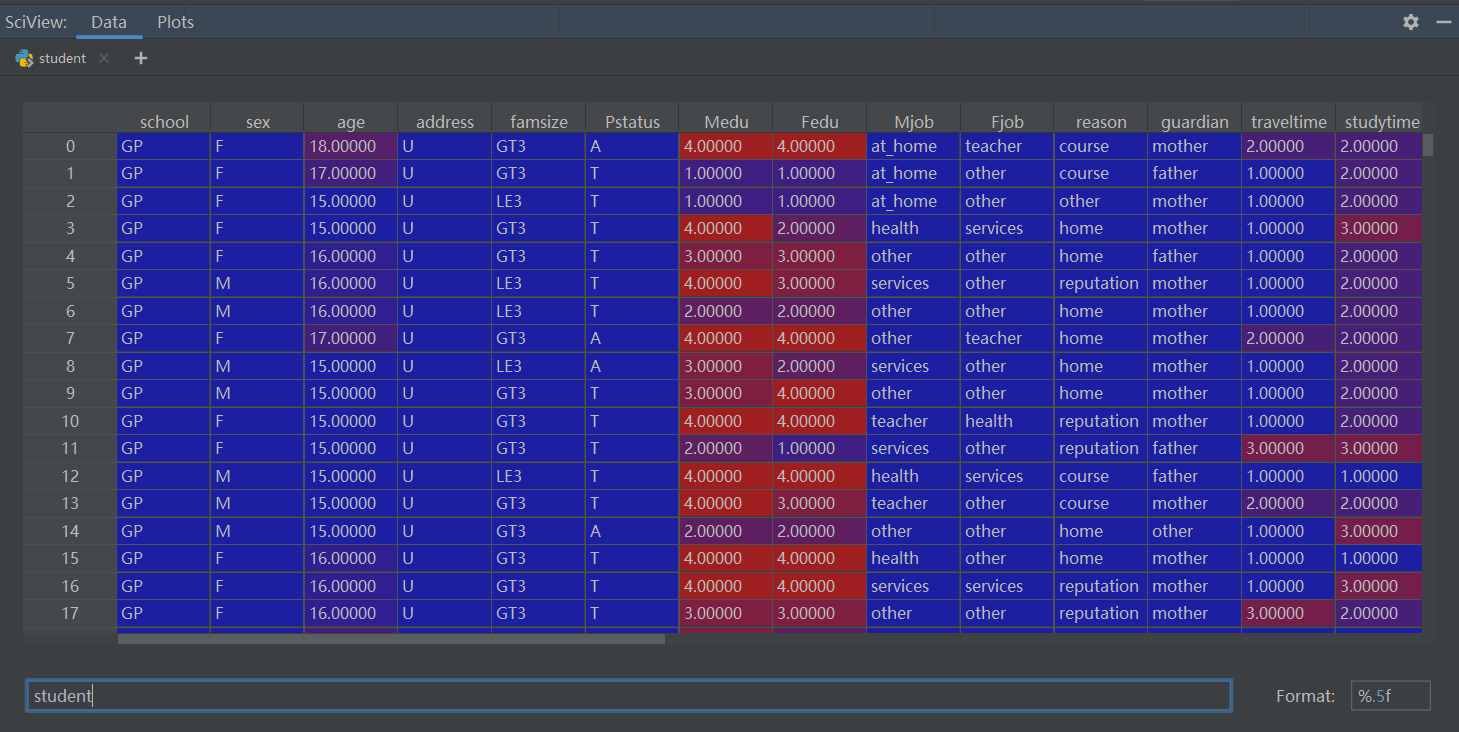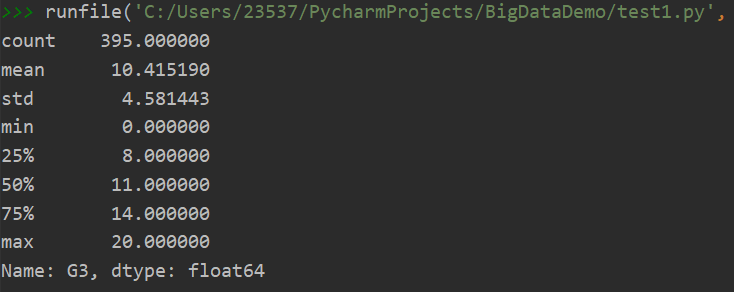### 3.2 利用图表分析属性

#### 3.2.1各分数段学生计数

  # 根据人数多少统计各分数段的学生人数

plt.show()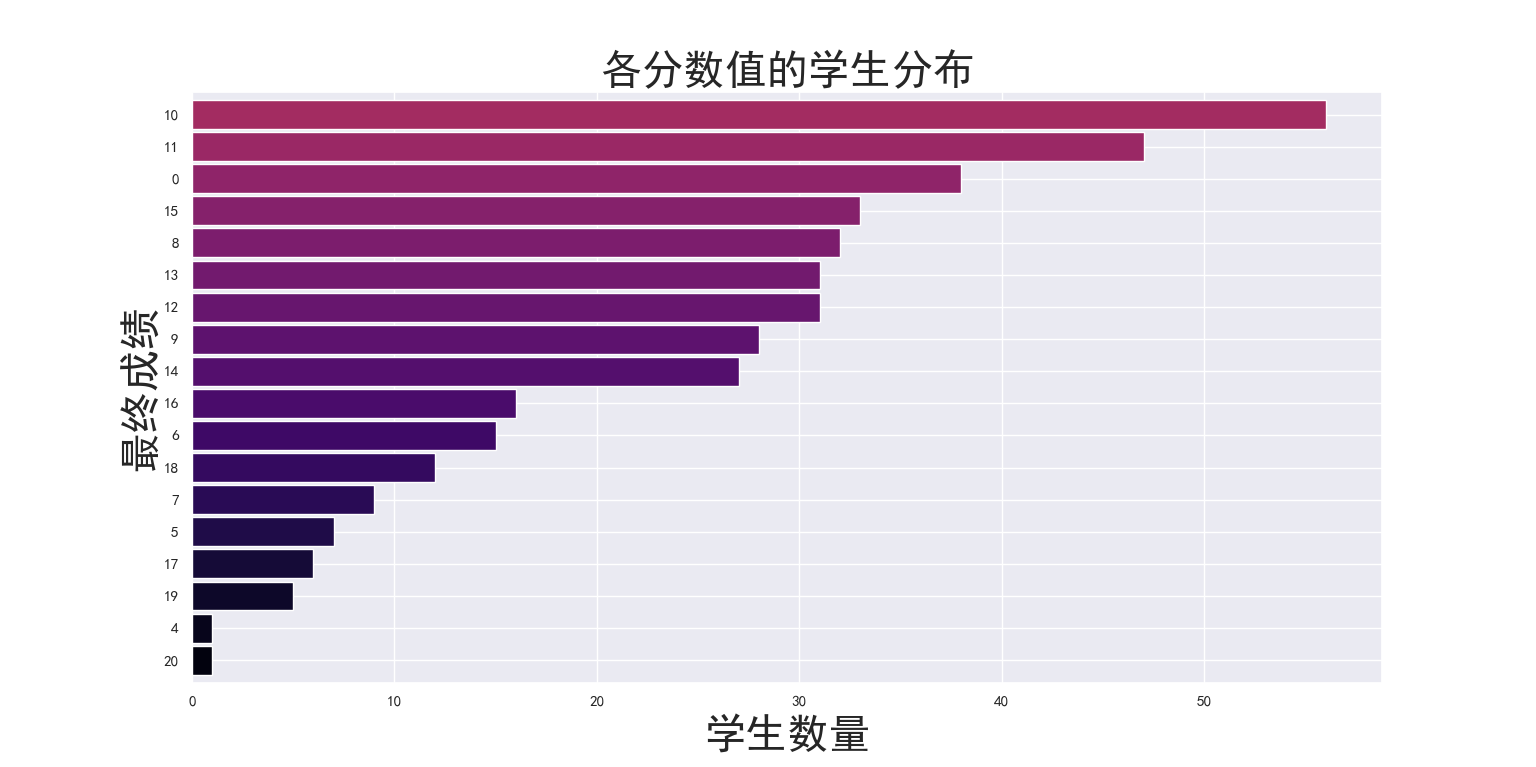#### 3.2.2 学生成绩分布直方图

3.2.1节中的图像并没有告诉我们有价值的信息，也许我们真正需要的是成绩分布直方图，即把成绩作为一个坐标轴，代码如下：

# 从低到高展示成绩分布图
plt.show()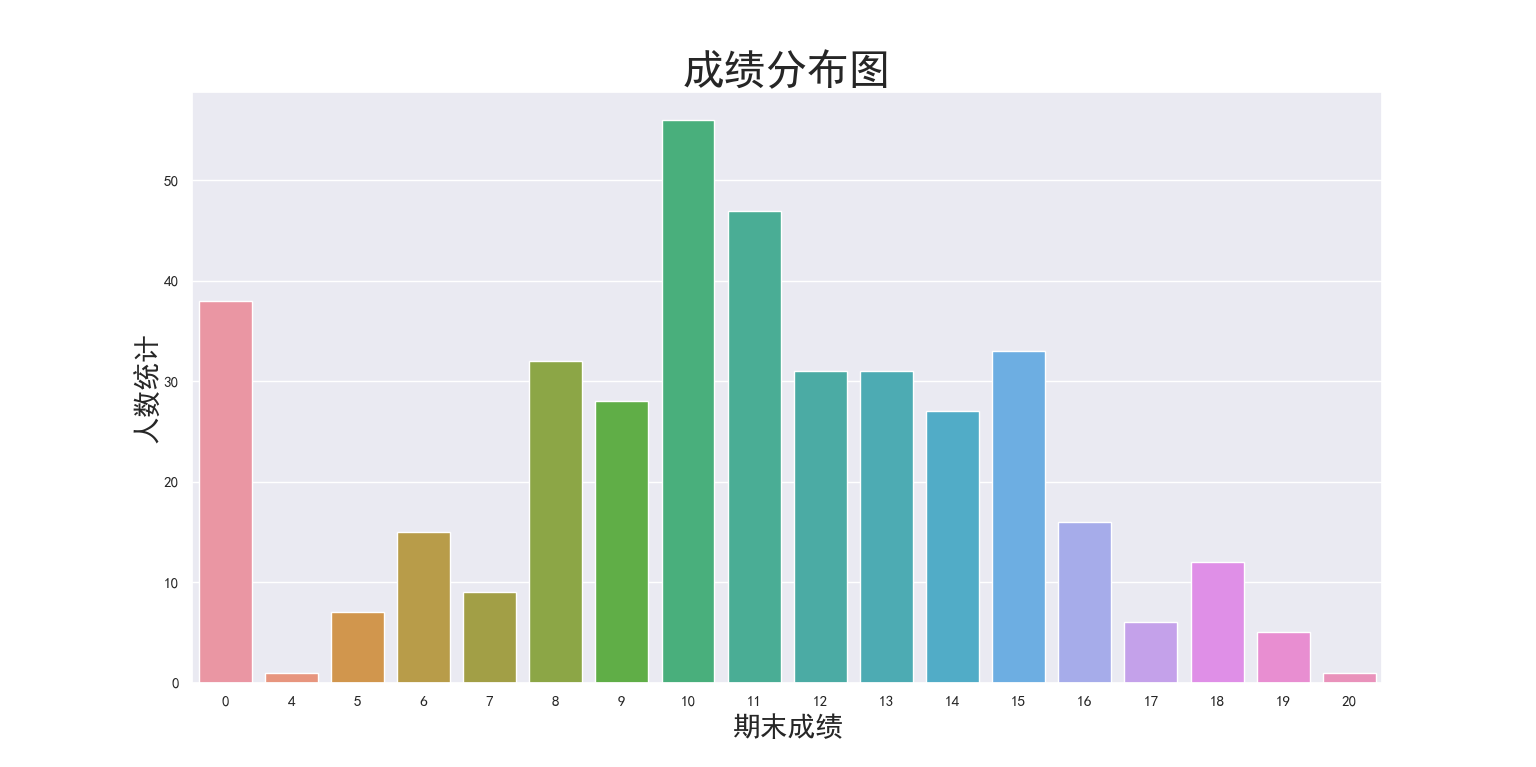#### 3.2.3 年龄因素分析

# 分析性别比例

male_studs = len(student[student['sex'] == 'M'])

female_studs = len(student[student['sex'] == 'F'])

print('男同学数量:',male_studs)

print('女同学数量:',female_studs)


# 分析年龄分布比例（曲线图）

age_distribution.axes.set_title('学生年龄分布图', fontsize=30)

age_distribution.set_xlabel('年龄', fontsize=20)

age_distribution.set_ylabel('比例', fontsize=20)

plt.show()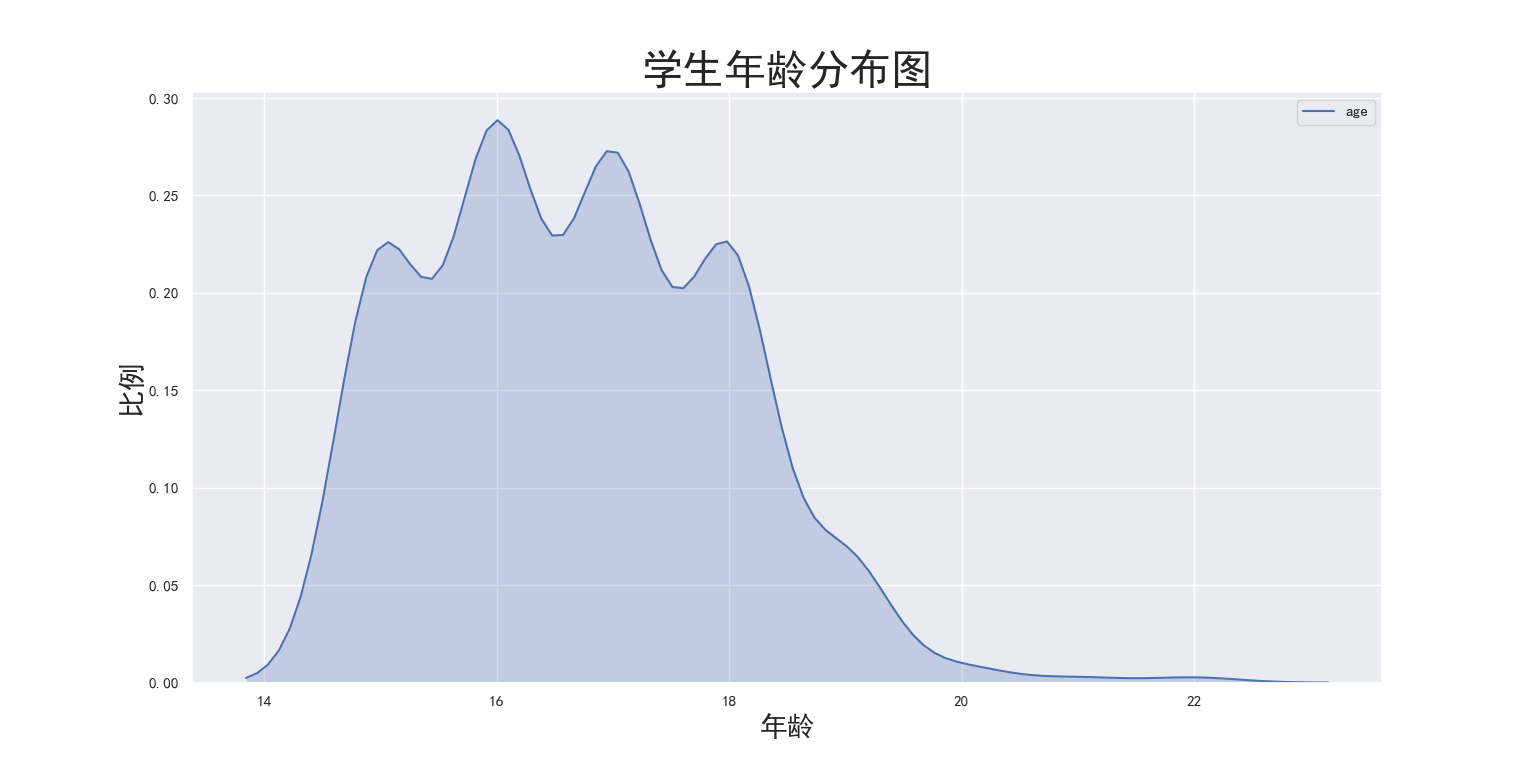# 分性别年龄分布图（柱状图）

age_distribution_sex = sns.countplot('age', hue='sex', data=student)

age_distribution_sex.axes.set_title('不同年龄段的学生人数', fontsize=30)

age_distribution_sex.set_xlabel('年龄', fontsize=30)

age_distribution_sex.set_ylabel('人数', fontsize=30)

plt.show()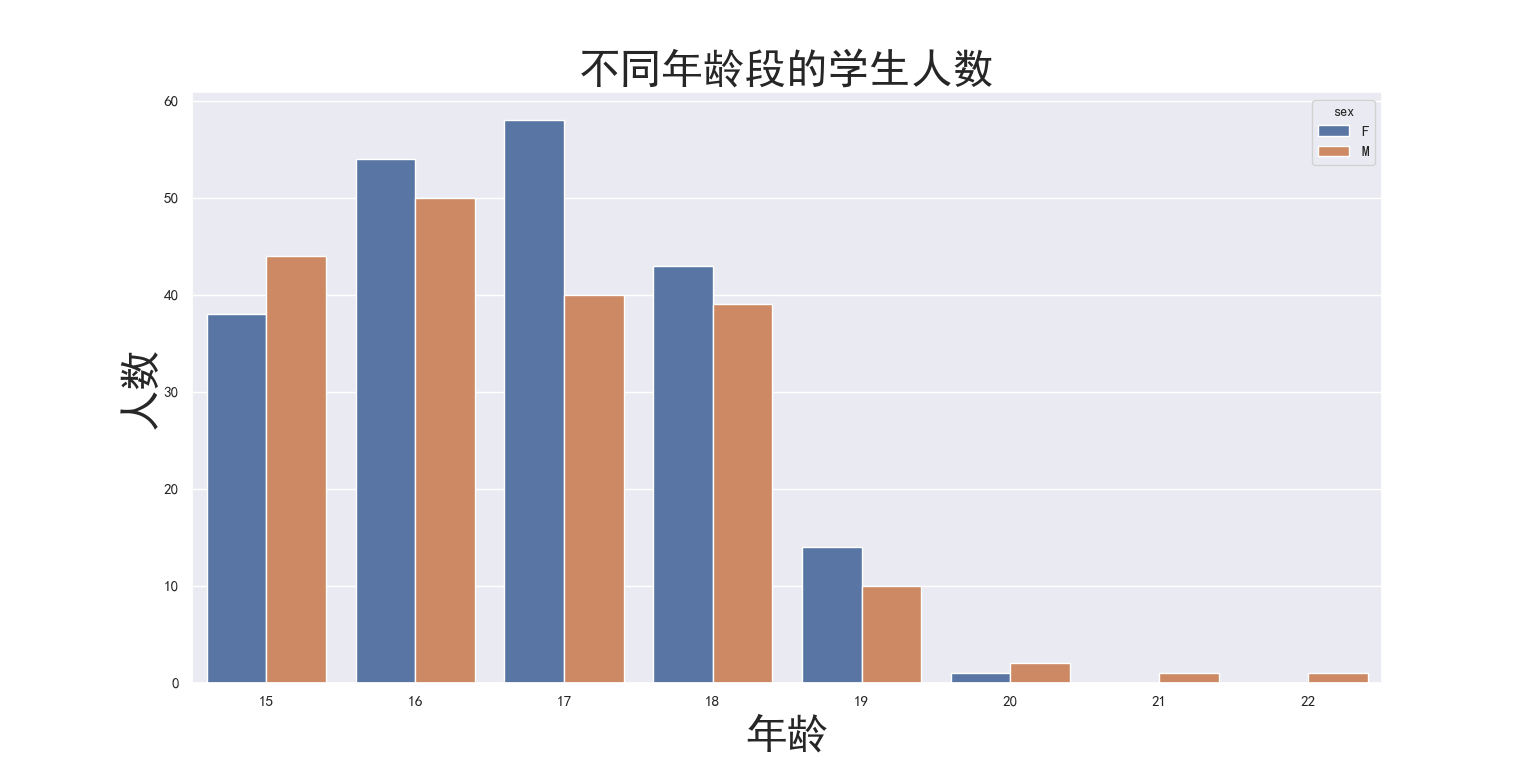# 各年龄段的成绩箱型图

plt.show()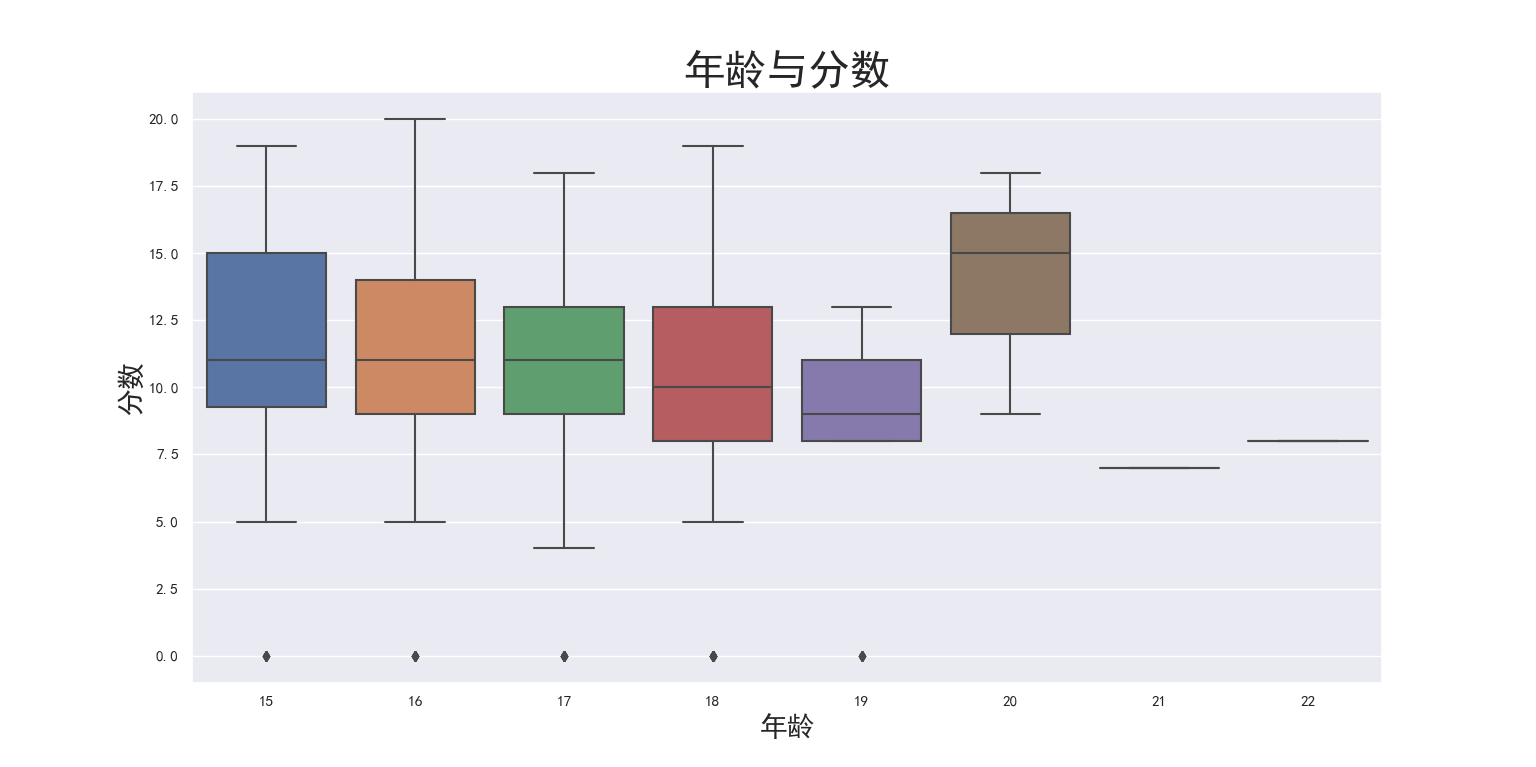# 各年龄段的成绩分布图

plt.show()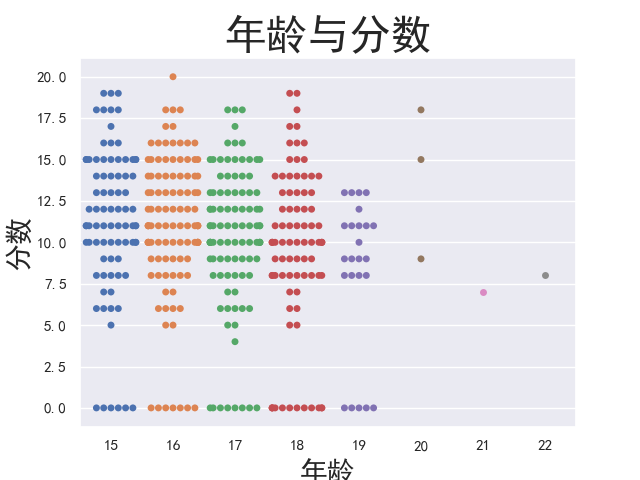#### 3.2.4 学生城乡因素分析

# 城乡学生计数

areas_countplot.axes.set_title('城乡学生', fontsize = 30)

areas_countplot.set_xlabel('家庭住址', fontsize = 20)

areas_countplot.set_ylabel('计数', fontsize = 20)

plt.show()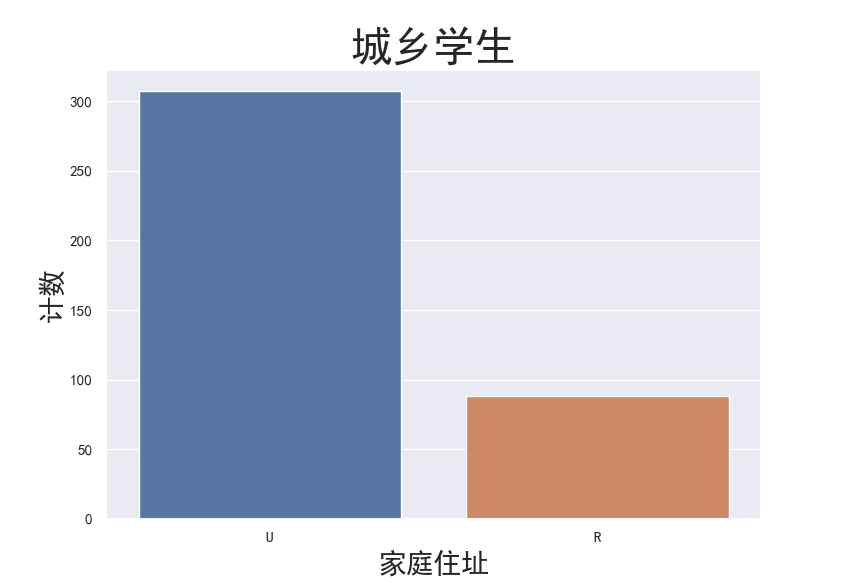​ 大部分学生来自城市，并且城乡比例约为3 ：1。但是城市学生一定获得了更好的成绩吗？还需要进一步分析，代码如下：
sns.kdeplot(student.loc[student['address'] == 'U', 'G3'], label='Urban', shade = True)

plt.title('城市学生获得了更好的成绩吗？', fontsize = 20)

plt.xlabel('分数', fontsize = 20)

plt.ylabel('占比', fontsize = 20)

plt.show()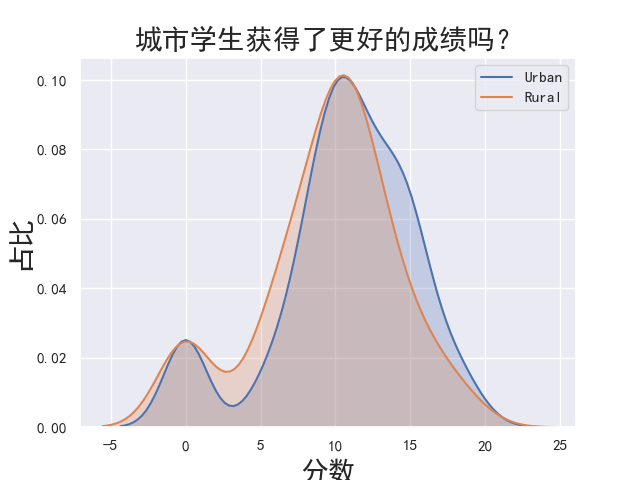### 3.3 各属性与成绩的相关性分析

#### 3.3.1相关性初步计算

student.corr()[‘G3’].sort_values()

\1.  failures   -0.360415

\2.  age     -0.161579

\3.  goout    -0.132791

\4.  traveltime  -0.117142

\5.  health    -0.061335

\6.  Dalc     -0.054660

\7.  Walc     -0.051939

\8.  freetime   0.011307

\9.  absences   0.034247

\10. famrel    0.051363

\11. studytime   0.097820

\12. Fedu     0.152457

\13. Medu     0.217147

\14. G1      0.801468

\15. G2      0.904868

\16. G3      1.000000

\17. Name: G3, dtype: float64


Occupation Label
Programmer 0
Data Scientist 1
Engineer 2

​ 标签编码的问题是整数的分配是随机的，并且每次运行函数时都会改变。 此外，模型可能会为更大的标签赋予更高的优先级。当我们只有2个唯一值时，可以使用标签编码。

Occupation Occupation_prog Occupation_ds Occupation_eng
Programmer 1 0 0
Data Scientist 0 1 0
Enginner 0 0 1

#### 3.3.2 编码后的相关性计算

​ 利用独热编码处理我们的数据集，然后再次寻找相关性，尽管G1和G2是学生的期末成绩，并且与期末成绩G3高度相关，但我们将其删除。没有G2和G1的情况下预测G3更加困难，但是这种预测更加有用，因为我们希望找到影响成绩的其他因素。代码如下：

# 选取G3属性值

labels = student['G3']

# 删除school，G1和G2属性

student = student.drop(['school', 'G1', 'G2'], axis='columns')

# 对离散变量进行独热编码

student = pd.get_dummies(student)

# 选取相关性最强的6个

most_correlated = student.corr().abs()['G3'].sort_values(ascending=False)

most_correlated = most_correlated[:6]

print(most_correlated)


\1.  G3       1.000000

\2.  failures    0.360415

\3.  Medu      0.217147

\4.  higher_yes   0.182465

\5.  higher_no   0.182465

\6.  age      0.161579

\7.  Fedu      0.152457

\8.  Name: G3, dtype: float64


### 3.4 高相关度属性对成绩的影响

#### 3.4.1 失败次数对成绩的影响

​ 代码如下：

# 失败次数成绩分布图

failures_swarmplot = sns.swarmplot(x=student['failures'],y=student['G3'])

failures_swarmplot.axes.set_title('失败次数少的学生分数更高吗？', fontsize = 30)

failures_swarmplot.set_xlabel('失败次数', fontsize = 20)

failures_swarmplot.set_ylabel('最终成绩', fontsize = 20)

plt.show()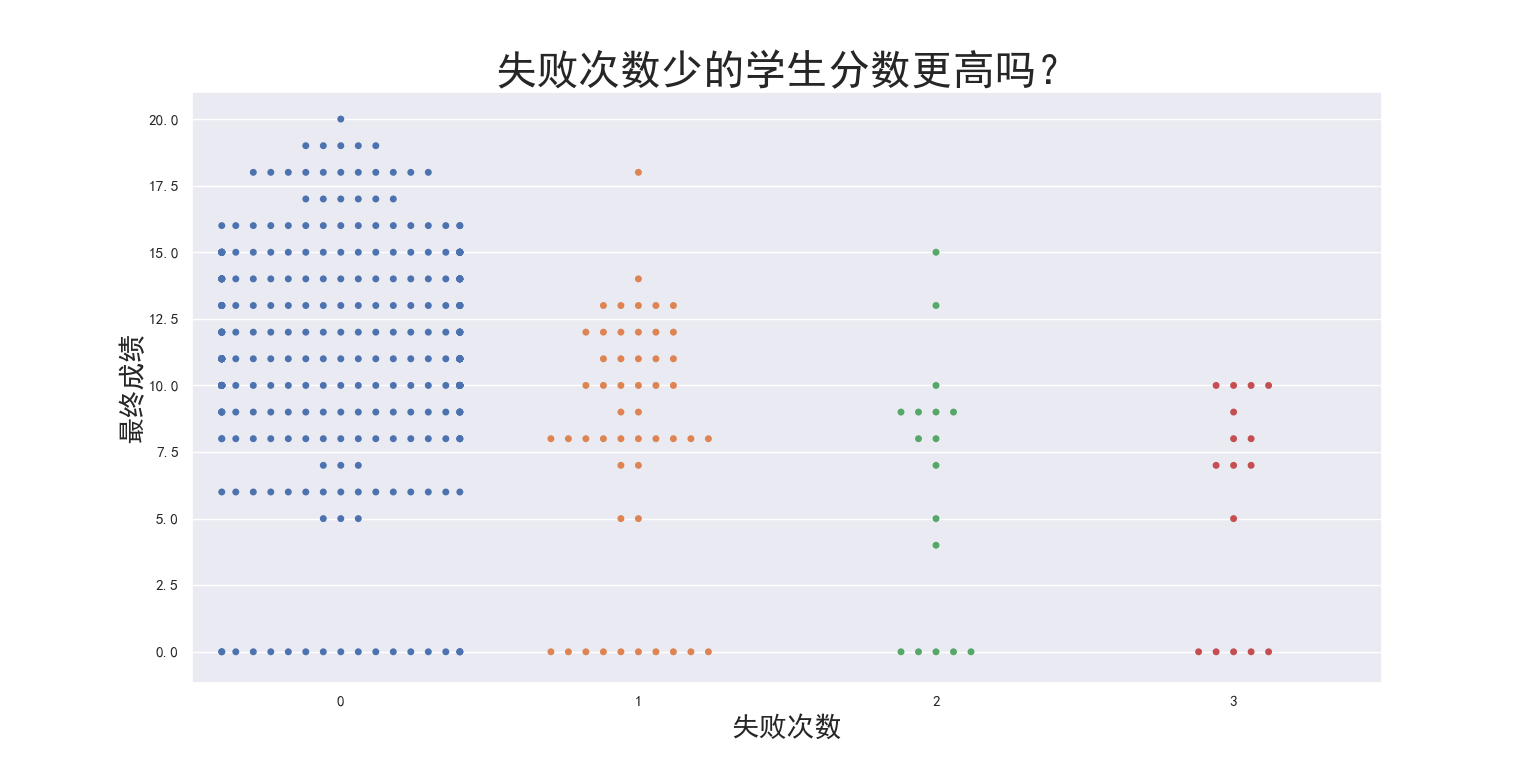​ 结果很明显，失败次数少的学生其成绩分布图更偏向于高分，可见失败次数少的学生更容易拿到高分，这或许是因为失败次数少的学生自信心更强。

#### 3.4.2 双亲受教育水平对成绩的影响

​ 代码如下：

# 双亲受教育水平的影响

family_ed = student['Fedu'] + student['Medu']

family_ed_boxplot = sns.boxplot(x=family_ed,y=student['G3'])

family_ed_boxplot.axes.set_title('双亲受教育水平的影响', fontsize = 30)

family_ed_boxplot.set_xlabel('家庭教育水平(Mother + Father)', fontsize = 20)

family_ed_boxplot.set_ylabel('最终成绩', fontsize = 20)

plt.show()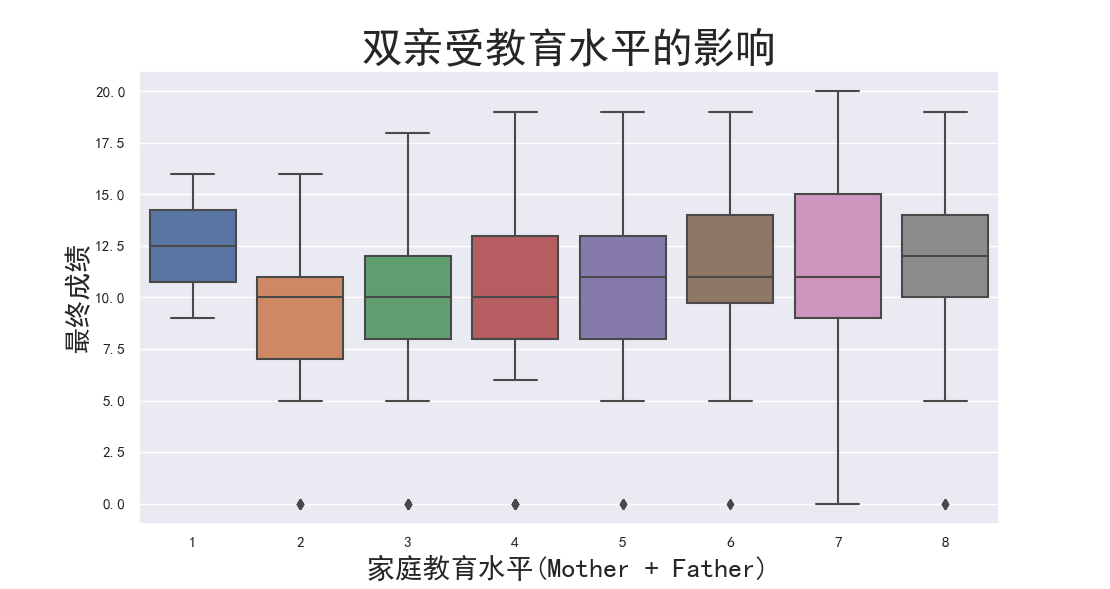​ 可见在一定范围内，随着家庭教育水平的提升，学生的平均成绩也有所提升，但是在图表的两极却出现了规律的之外的东西，家庭受教育水平最低的学生，最终成绩高于不少其他水平的家庭，而家庭受教育水平最高的家庭，孩子的成绩却不是最高。原因暂时不明，且不排除数据错误的可能。

#### 3.4.3 学生升学意愿对成绩的影响

​ 代码如下：

# 学生自己的升学意志对成绩的影响

personal_wish = sns.boxplot(x = student['higher_yes'], y=student['G3'])

personal_wish.axes.set_title('学生升学意愿对成绩的影响', fontsize = 30)

personal_wish.set_xlabel('更高级的教育 (1 = 是)', fontsize = 20)

personal_wish.set_ylabel('最终成绩', fontsize = 20)

plt.show()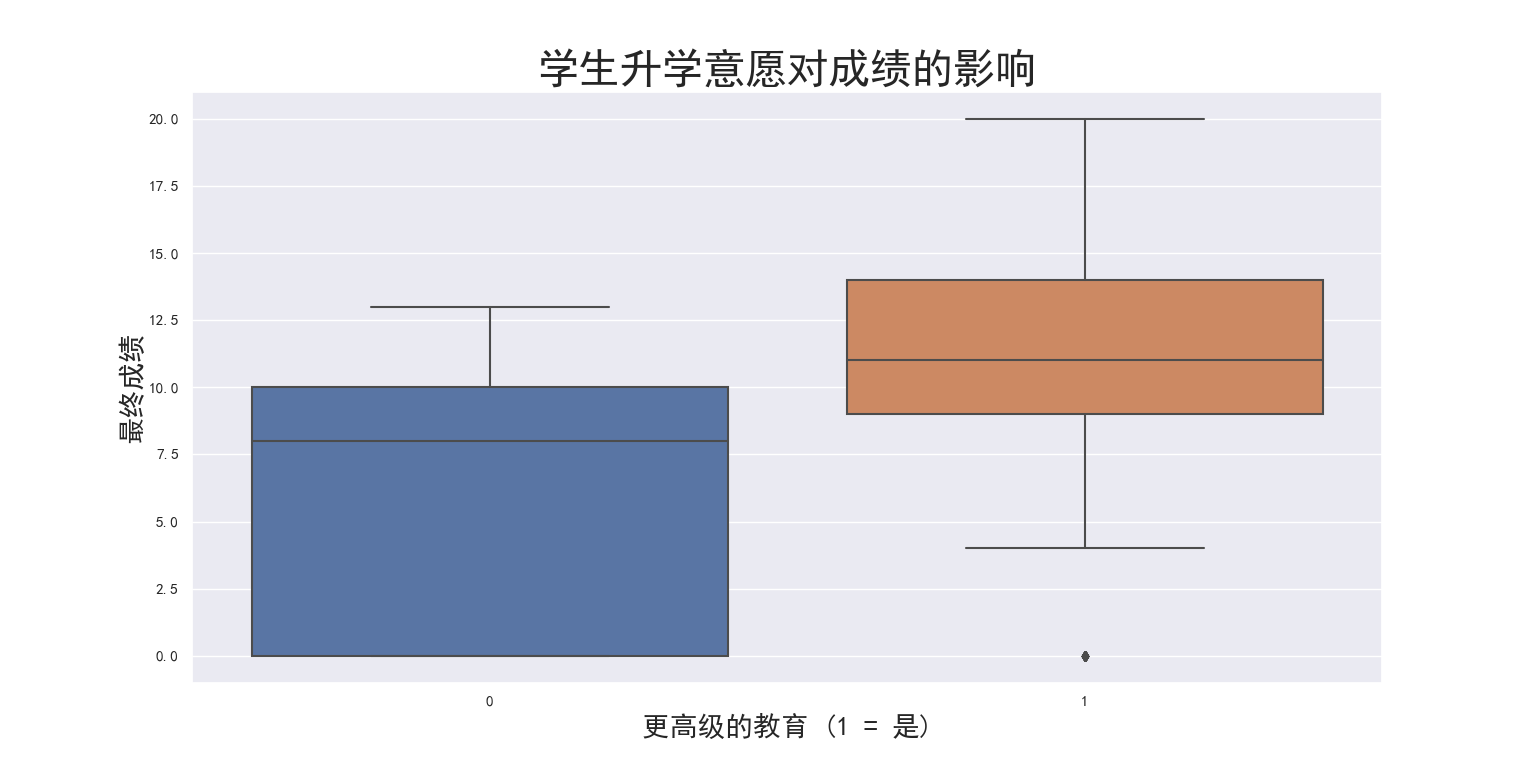### 3.5 大数据模型

#### 3.5.1 数据分割

X_train, X_test, y_train, y_test = train_test_split(student, labels, test_size = 0.25, random_state=42)


#### 3.5.2 训练之前的准备工作

# 计算平均绝对误差和均方根误差

# MAE-平均绝对误差

# RMSE-均方根误差

def evaluate_predictions(predictions, true):

mae = np.mean(abs(predictions - true))

rmse = np.sqrt(np.mean((predictions - true) ** 2))

return mae, rmse

# 求中位数

median_pred = X_train['G3'].median()

# 所有中位数的列表

median_preds = [median_pred **for** _ **in** range(len(X_test))]

# 存储真实的G3值以传递给函数

true = X_test['G3']

# 展示基准的原始指标

mb_mae, mb_rmse = evaluate_predictions(median_preds, true)

print('Median Baseline MAE: {:.4f}'.format(mb_mae))

print('Median Baseline RMSE: {:.4f}'.format(mb_rmse))


Median Baseline MAE: 3.7879

Median Baseline RMSE: 4.8252

#### 3.5.3 预测模型训练

# 通过训练集训练和测试集测试来生成多个线性模型
def evaluate(X_train, X_test, y_train, y_test):
# 模型名称
model_name_list = ['Linear Regression', 'ElasticNet Regression',
'Random Forest', 'Extra Trees', 'SVM',
X_train = X_train.drop('G3', axis='columns')
X_test = X_test.drop('G3', axis='columns')

# 实例化模型
model1 = LinearRegression()
model2 = ElasticNet(alpha=1.0, l1_ratio=0.5)
model3 = RandomForestRegressor(n_estimators=100)
model4 = ExtraTreesRegressor(n_estimators=100)
model5 = SVR(kernel='rbf', degree=3, C=1.0, gamma='auto')

# 结果数据框
results = pd.DataFrame(columns=['mae', 'rmse'], index = model_name_list)

# 每种模型的训练和预测
for i, model in enumerate([model1, model2, model3, model4, model5, model6]):
model.fit(X_train, y_train)
predictions = model.predict(X_test)

# 误差标准
mae = np.mean(abs(predictions - y_test))
rmse = np.sqrt(np.mean((predictions - y_test) ** 2))

# 将结果插入结果框
model_name = model_name_list[i]
results.loc[model_name, :] = [mae, rmse]

# 中值基准度量
baseline = np.median(y_train)
baseline_mae = np.mean(abs(baseline - y_test))
baseline_rmse = np.sqrt(np.mean((baseline - y_test) ** 2))

results.loc['Baseline', :] = [baseline_mae, baseline_rmse]

return results
results = evaluate(X_train, X_test, y_train, y_test)
print(results)


Linear Regression 3.41954 4.27557
ElasticNet Regression 3.62976 4.58258
Random Forest 3.04111 3.77742
Extra Trees 3.14586 4.05109
SVM 3.50899 4.38375
Baseline 3.78788 4.82523

## 4. 分析与总结

# 找出最合适的模型
plt.figure(figsize=(12, 8))

# 平均绝对误差
ax =  plt.subplot(1, 2, 1)
results.sort_values('mae', ascending = True).plot.bar(y = 'mae', color = 'b', ax = ax, fontsize=20)
plt.title('平均绝对误差', fontsize=20)
plt.ylabel('MAE', fontsize=20)

# 均方根误差
ax = plt.subplot(1, 2, 2)
results.sort_values('rmse', ascending = True).plot.bar(y = 'rmse', color = 'r', ax = ax, fontsize=20)
plt.title('均方根误差', fontsize=20)
plt.ylabel('RMSE',fontsize=20)
plt.tight_layout()
plt.show()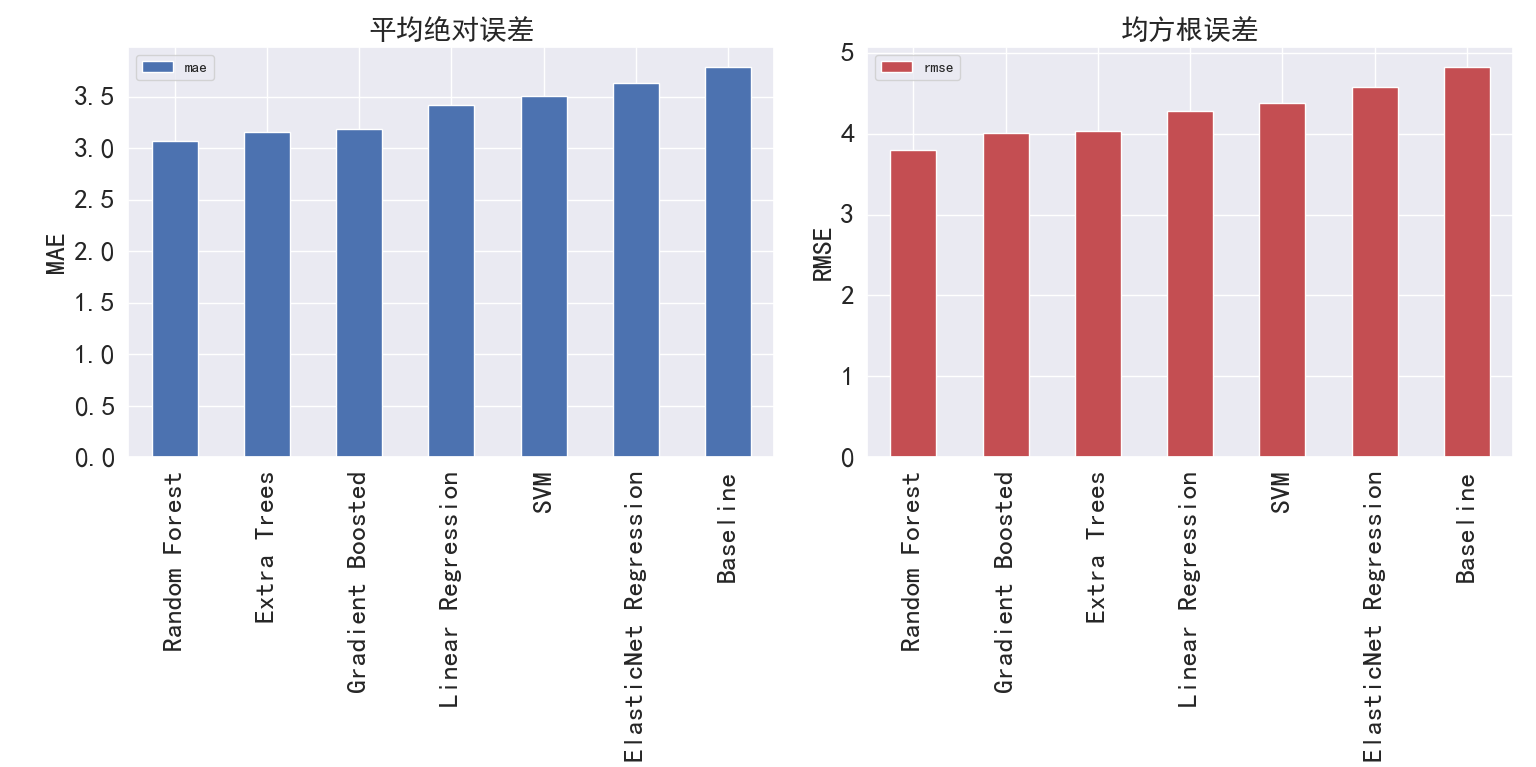# 保存线性回归模型*

model = LinearRegression()

model.fit(X_train, y_train)

filename = 'LR_Model'

pickle.dump(model, open(filename, 'wb'))


se’, color = ‘r’, ax = ax, fontsize=20)
plt.title(‘均方根误差’, fontsize=20)
plt.ylabel(‘RMSE’,fontsize=20)
plt.tight_layout()
plt.show()



[外链图片转存中...(img-737XOIuU-1576926060105)]

<center>图4-1 各模型误差柱状图</center>

*

python
# 保存线性回归模型*

model = LinearRegression()

model.fit(X_train, y_train)

filename = 'LR_Model'

pickle.dump(model, open(filename, 'wb'))
`©️2019 CSDN 皮肤主题: 大白 设计师: CSDN官方博客点击重新获取扫码支付